Question

Process Control

1. Both tanks are perfectly mixed.

2. Both tanks operate at constant volume, V and V.

3. The fluid density P is constant.

4. In the first tank, component A is consumed according to:

RA = -kCA

where k is a known constant. There is no chemical reaction in the second tank.Write

a. Write dynamic component A balances for both tanks. (Do not write any energy balances.)Eliminate total mass balances (if you wrote them), q2, and the rate equation, so that you onlyhave two equations for:

\frac{d c_{A 2}}{d t}=f_{2}\left(q, q_{3}, c_{A 1}, c_{A 2}, c_{A 3}\right)

b. Perform a degree of freedom analysis on your model. The variables should include:

\frac{d c_{A 1}}{d t}=f_{1}\left(q, c_{A i}, c_{A 1}\right)

q, q_{3}, c_{A i}, c_{A 1}, c_{A 2}, c_{A 3} \text {. Determine the model inputs and model outputs. Also assign DVs }

and MVs.

c. Linearize your model equations, and convert them to perturbation variables.

d. Take the Laplace transform of the linearized equation for dc,, (t)/dt from part c. You do not need to take the Laplace transform of the equation for dc', (t)/dtAlA2

e. Show that:

c_{A 1}^{\prime}(s)=G_{1}(s) c_{A i}^{\prime}(s)+G_{2}(s) q^{\prime}(s)

f. For G2 (s), find the steady-state gain and all time constants.

g. In part c, you linearized the model equations. These can be written in the state-space form:

\dot{x}=\left[\begin{array}{l} \dot{c}_{A 1}^{\prime} \\ \dot{c}_{A 2}^{\prime} \end{array}\right]=A x+B u+E d

Find the matrix A.Verified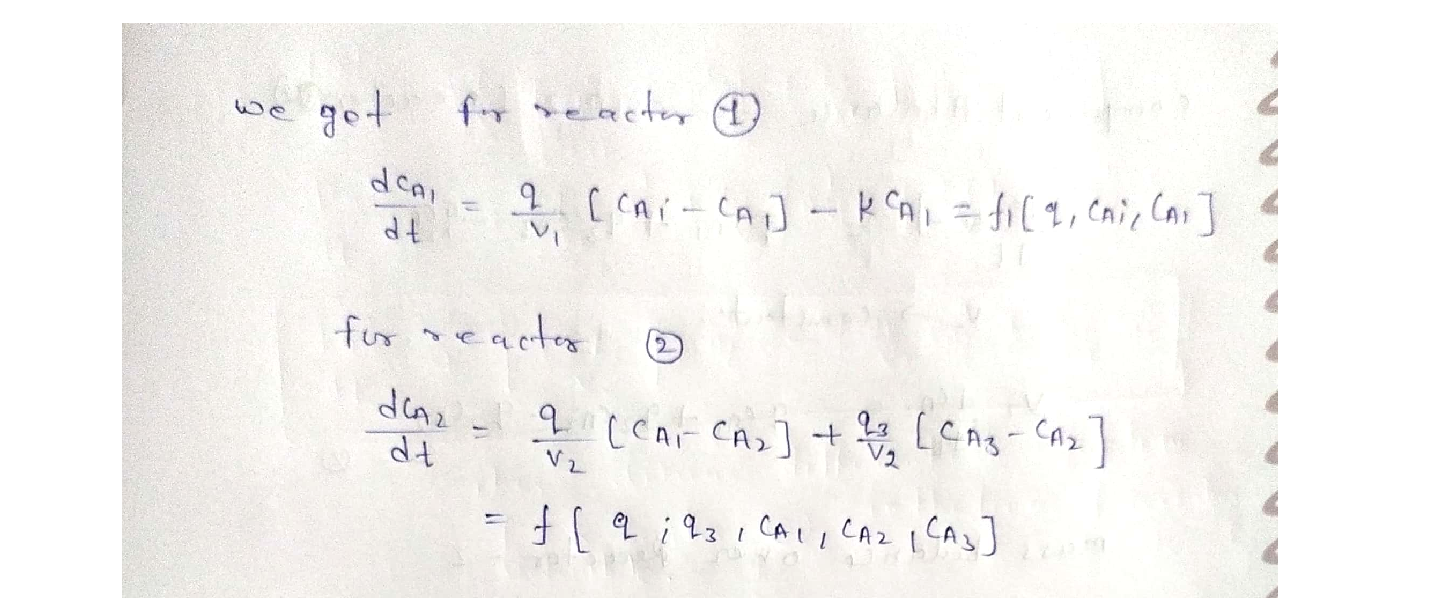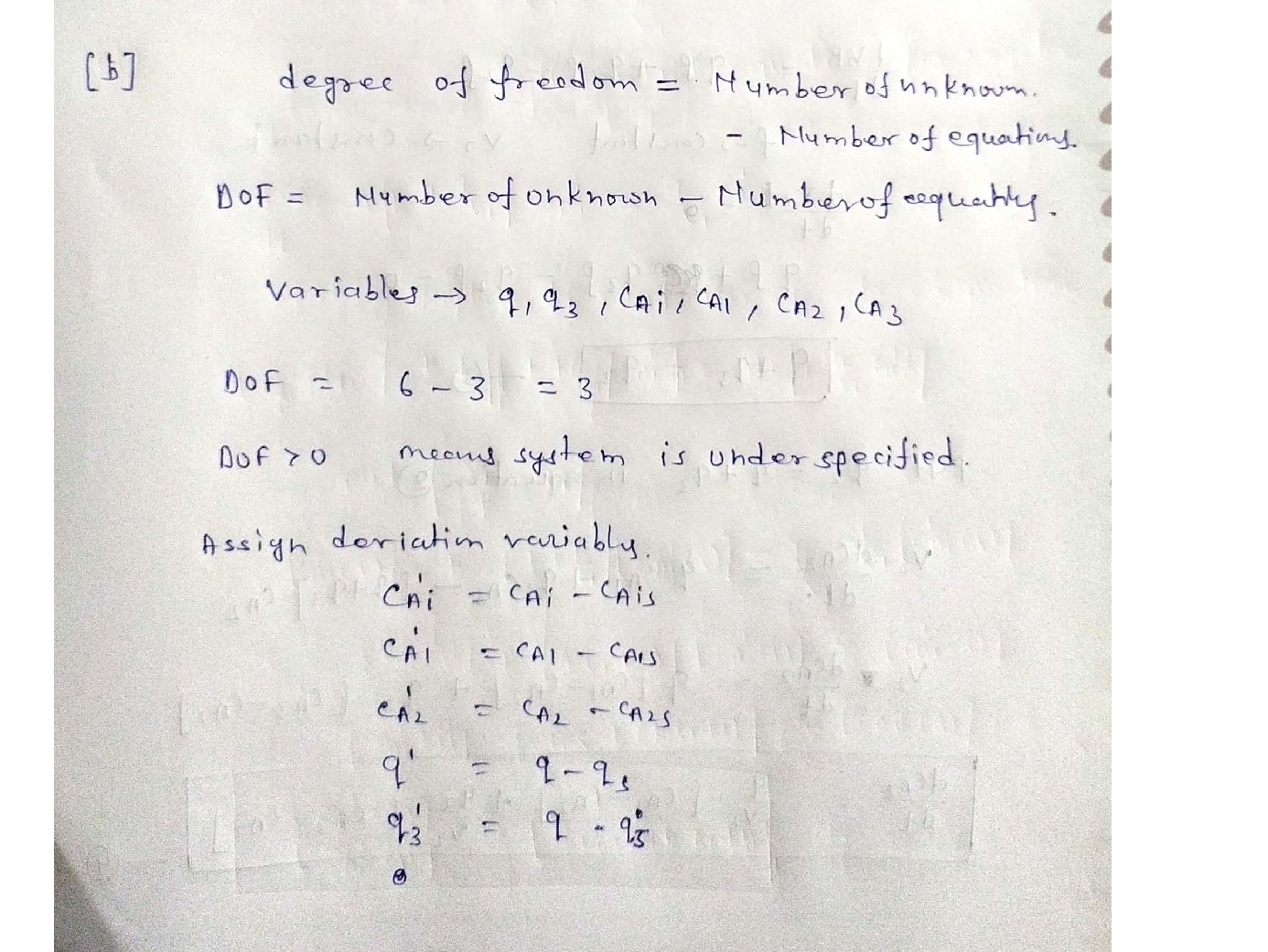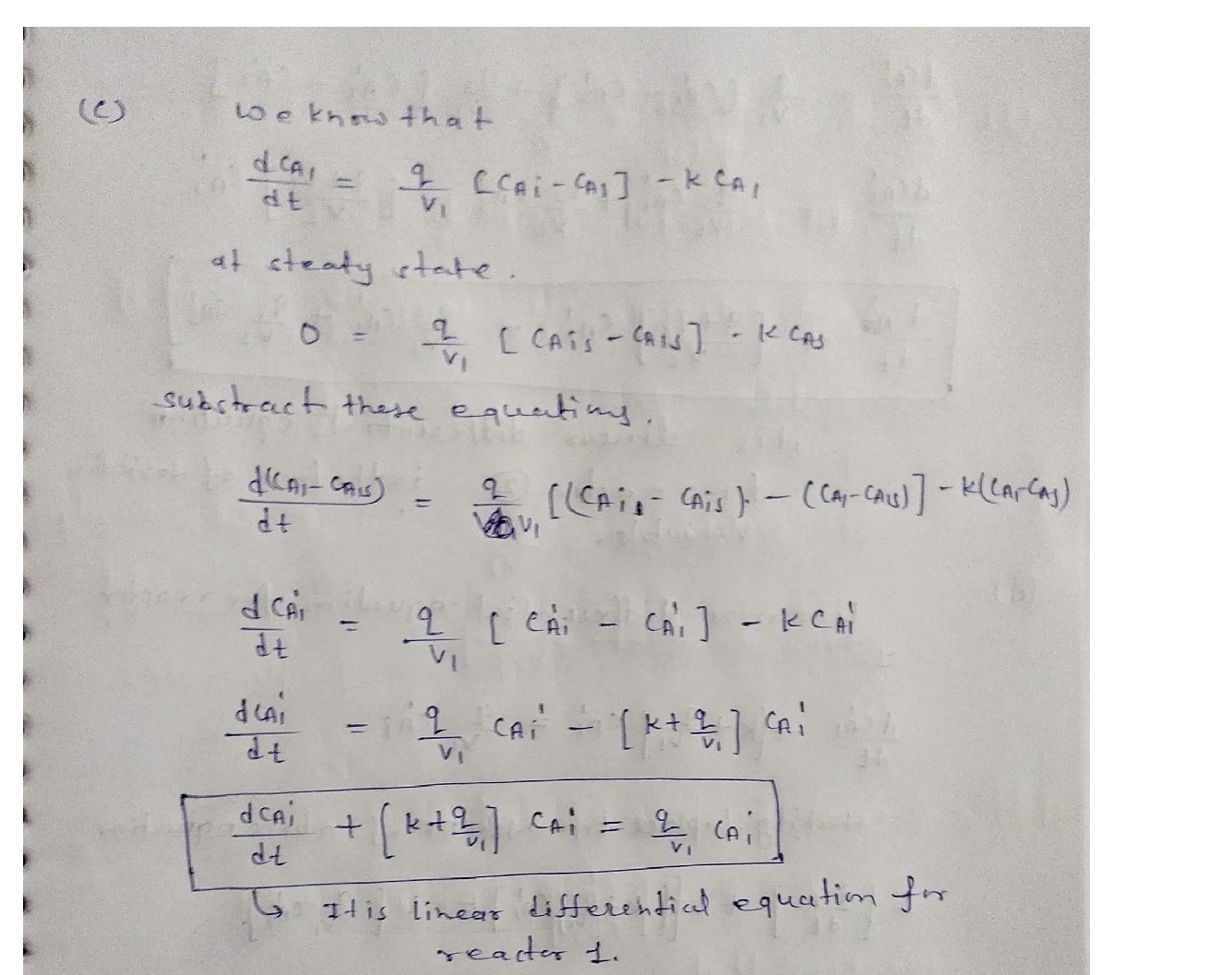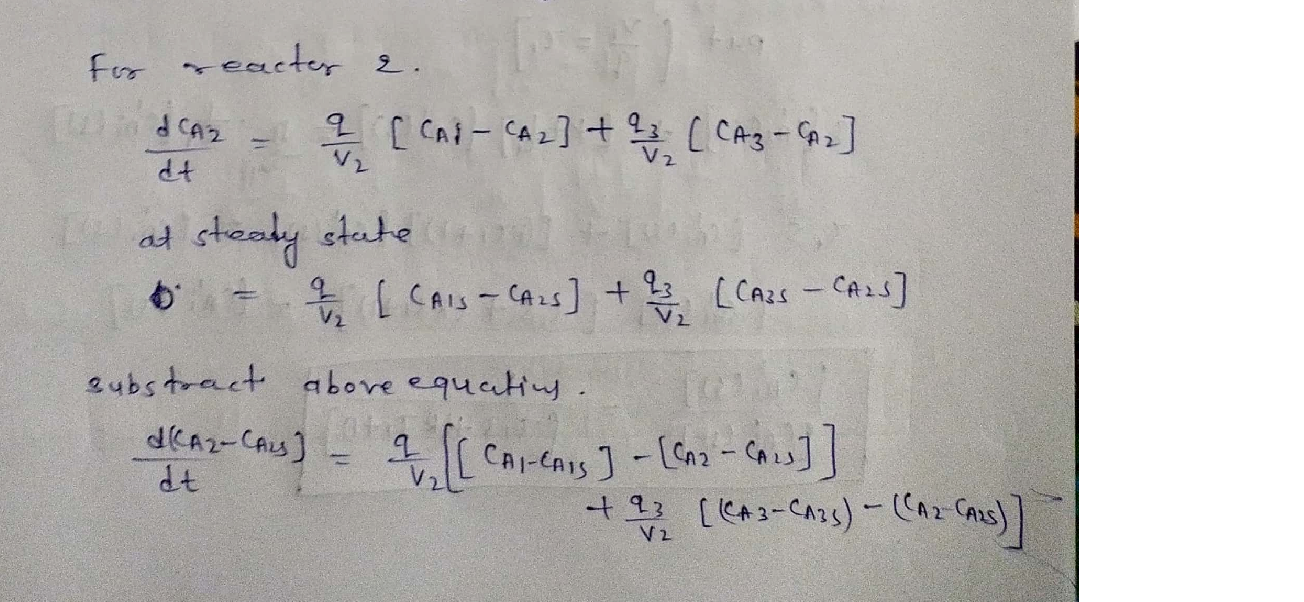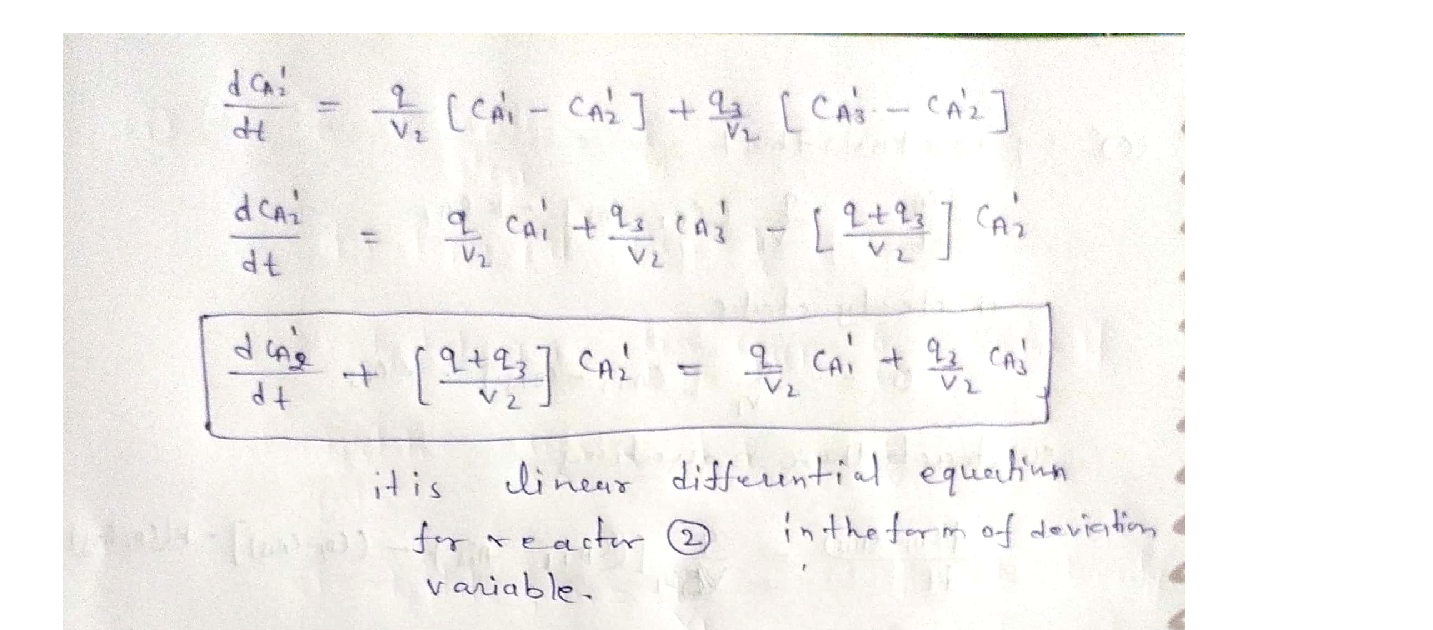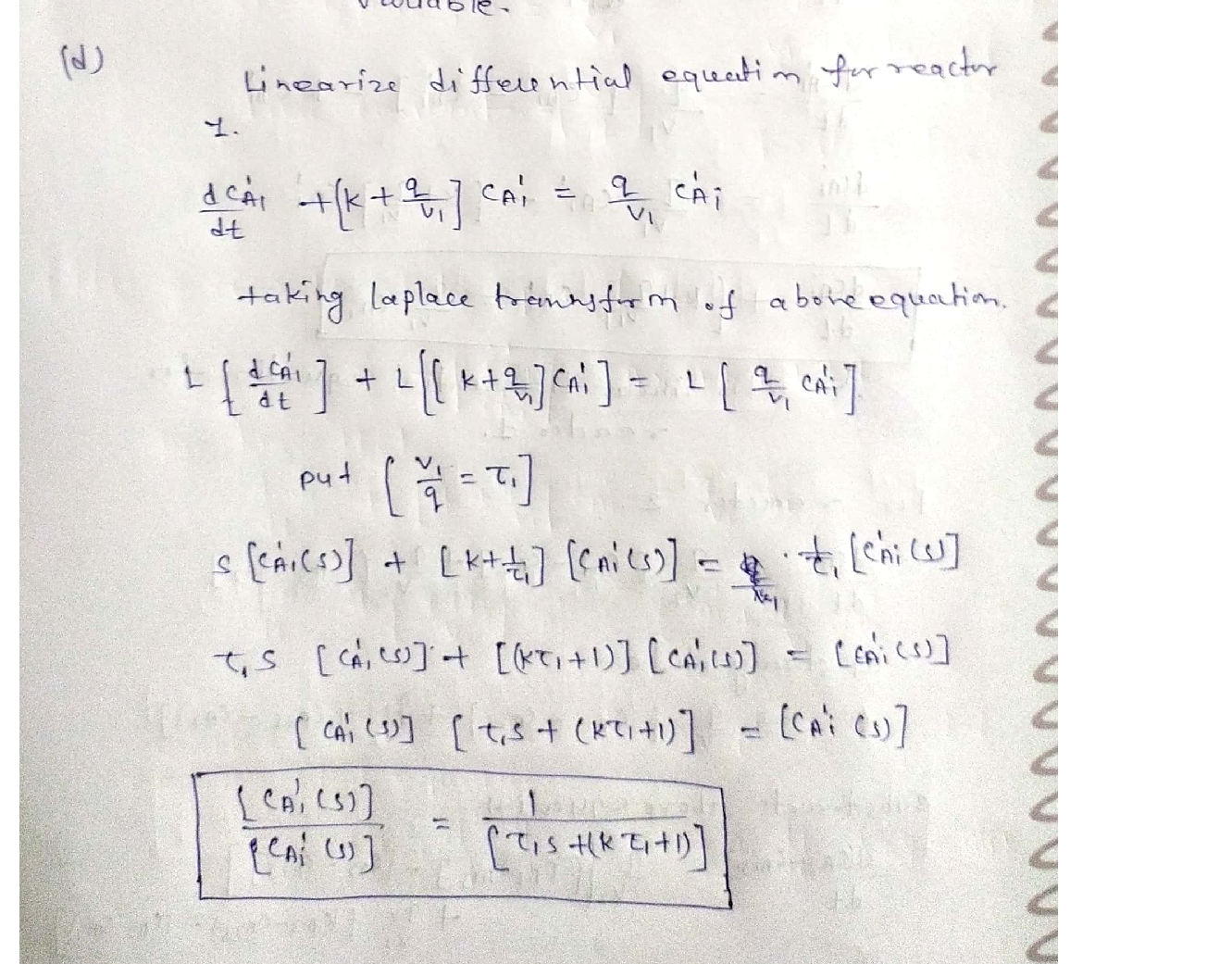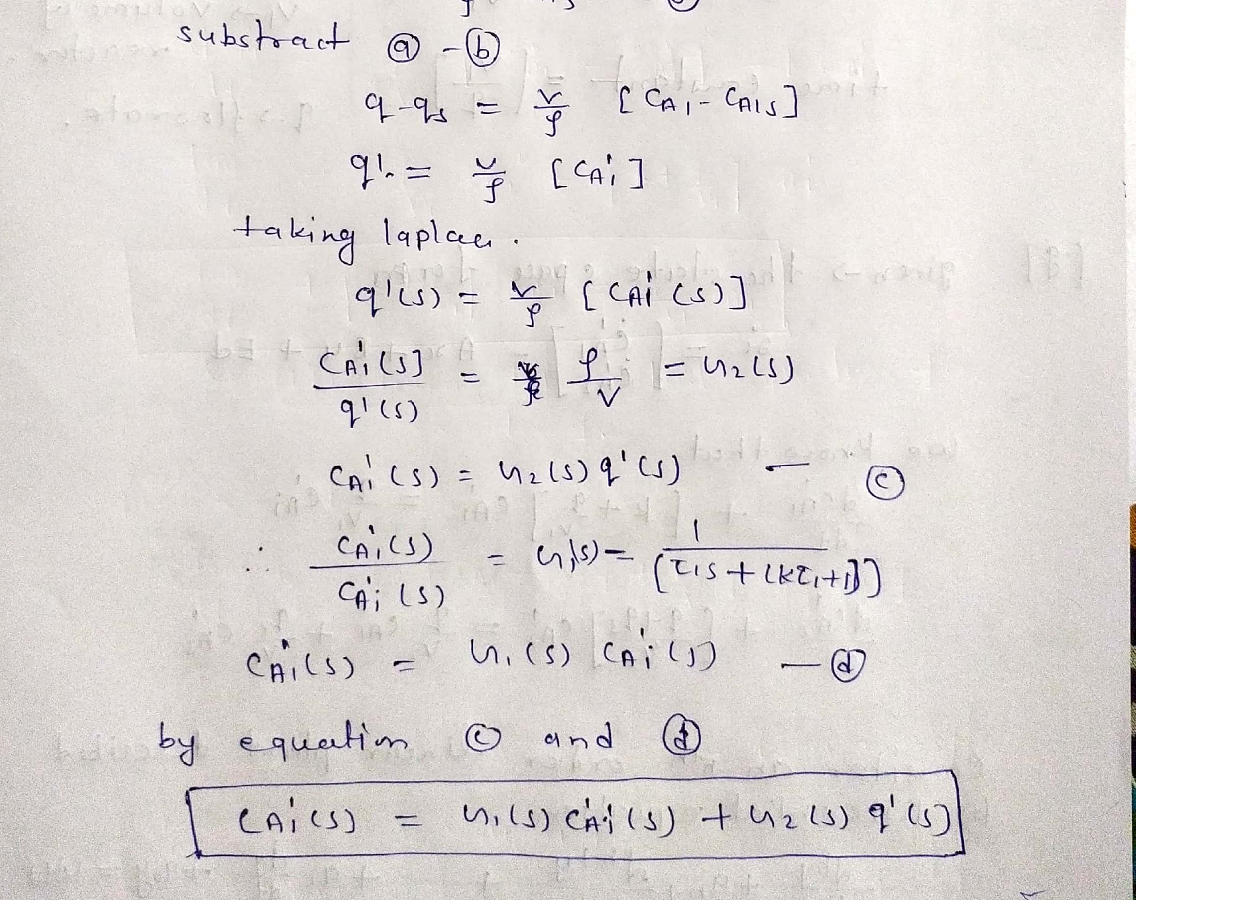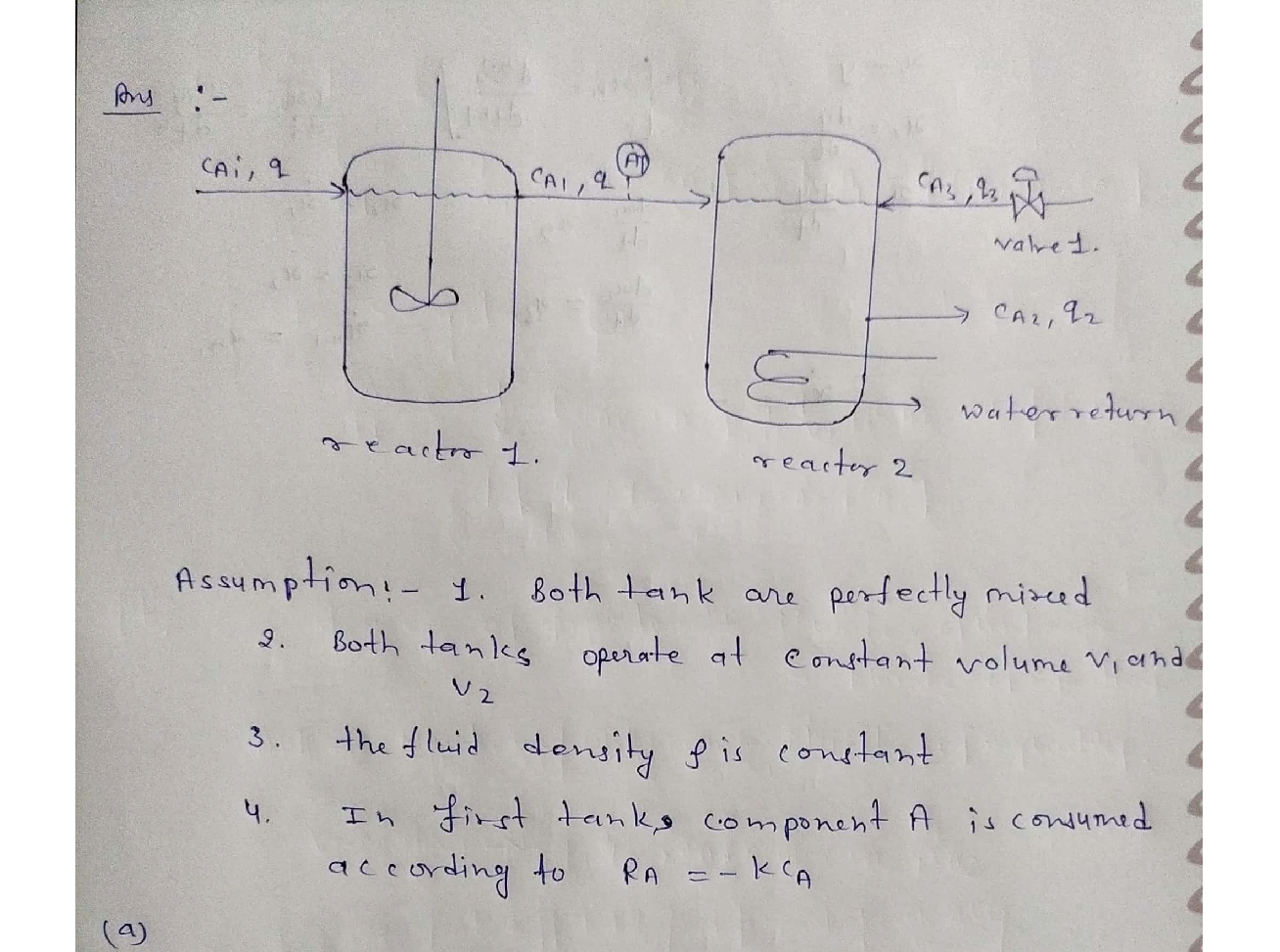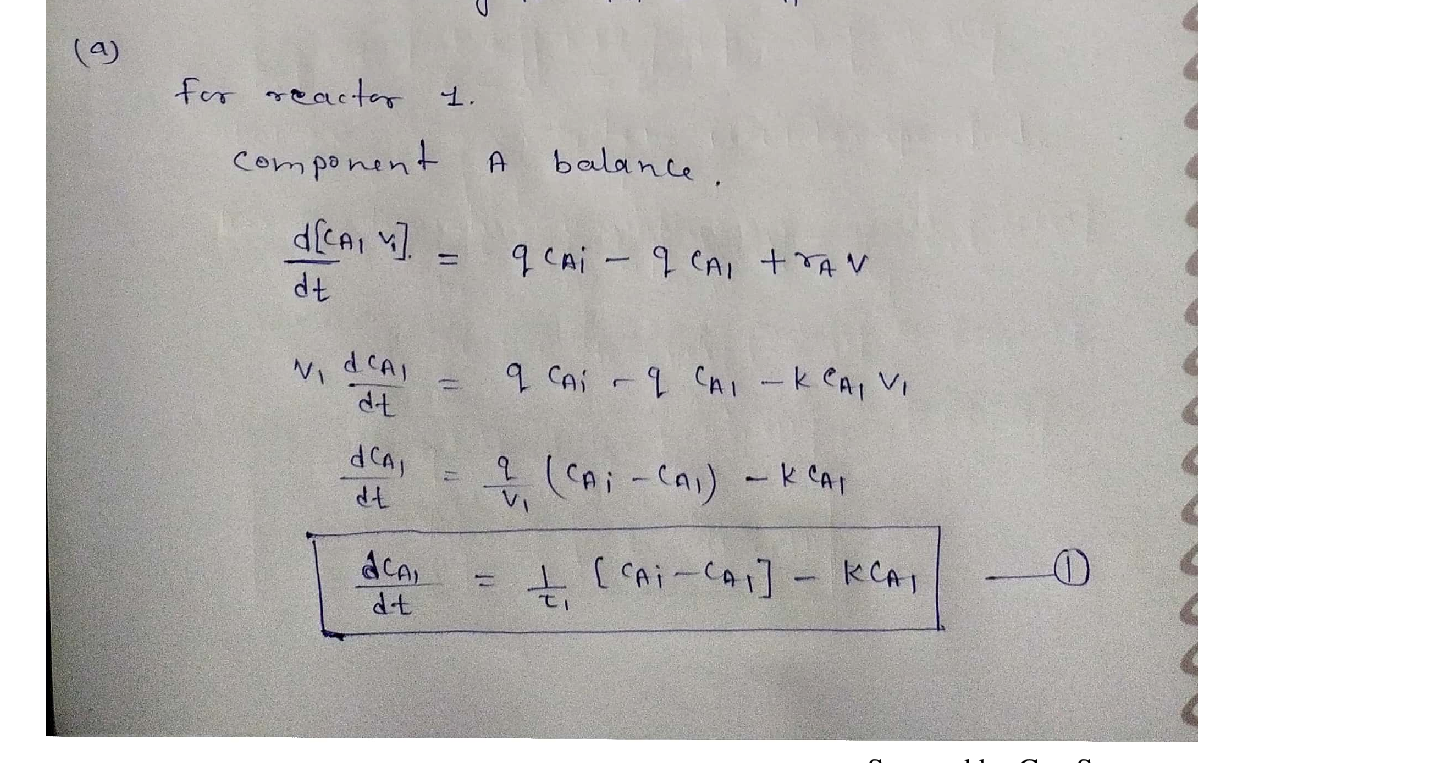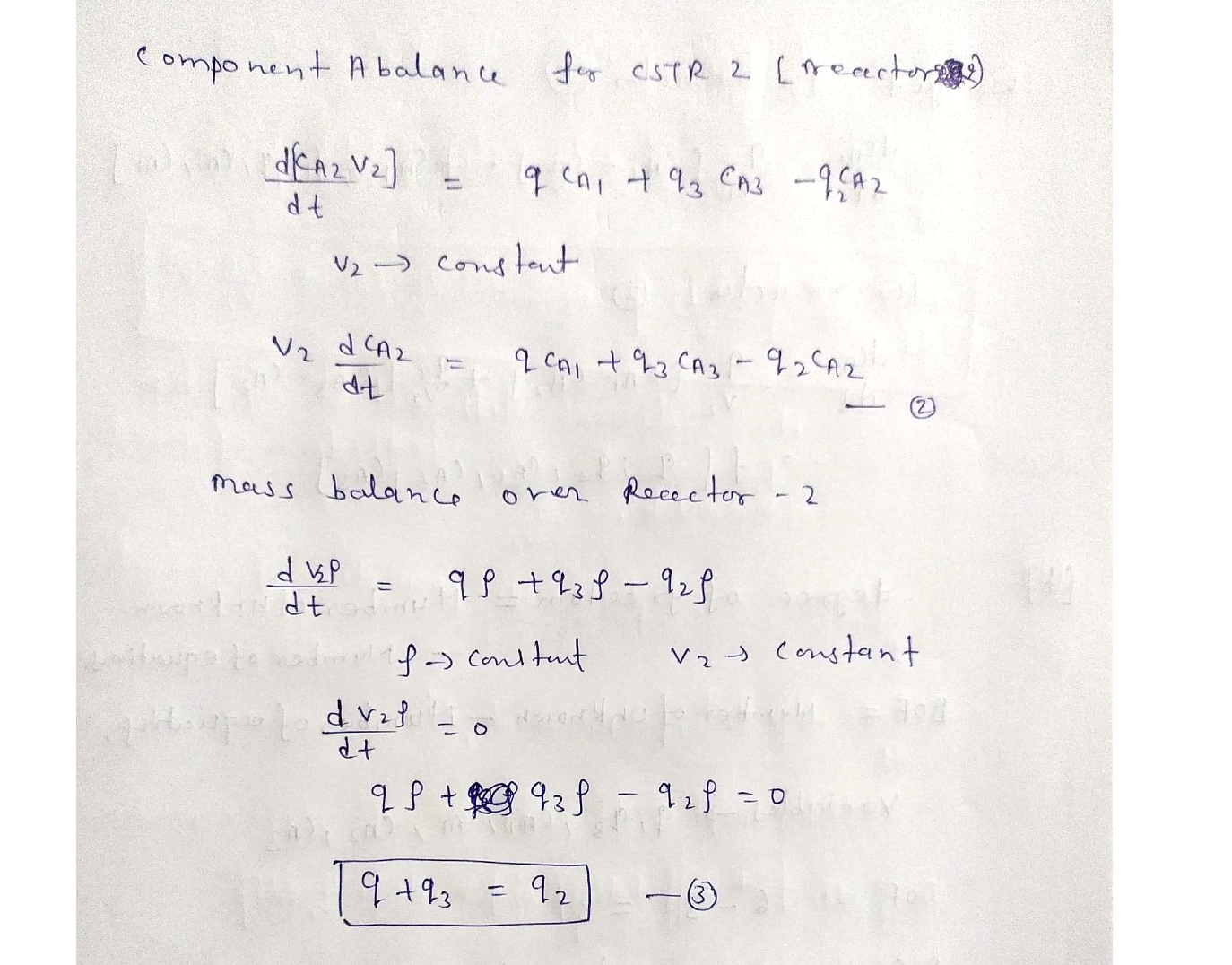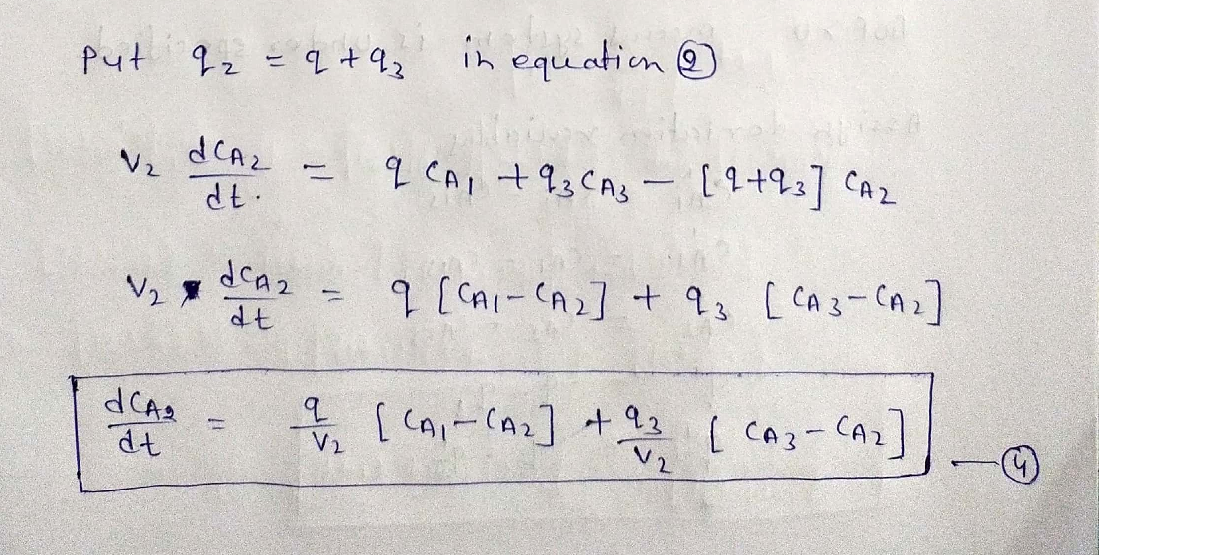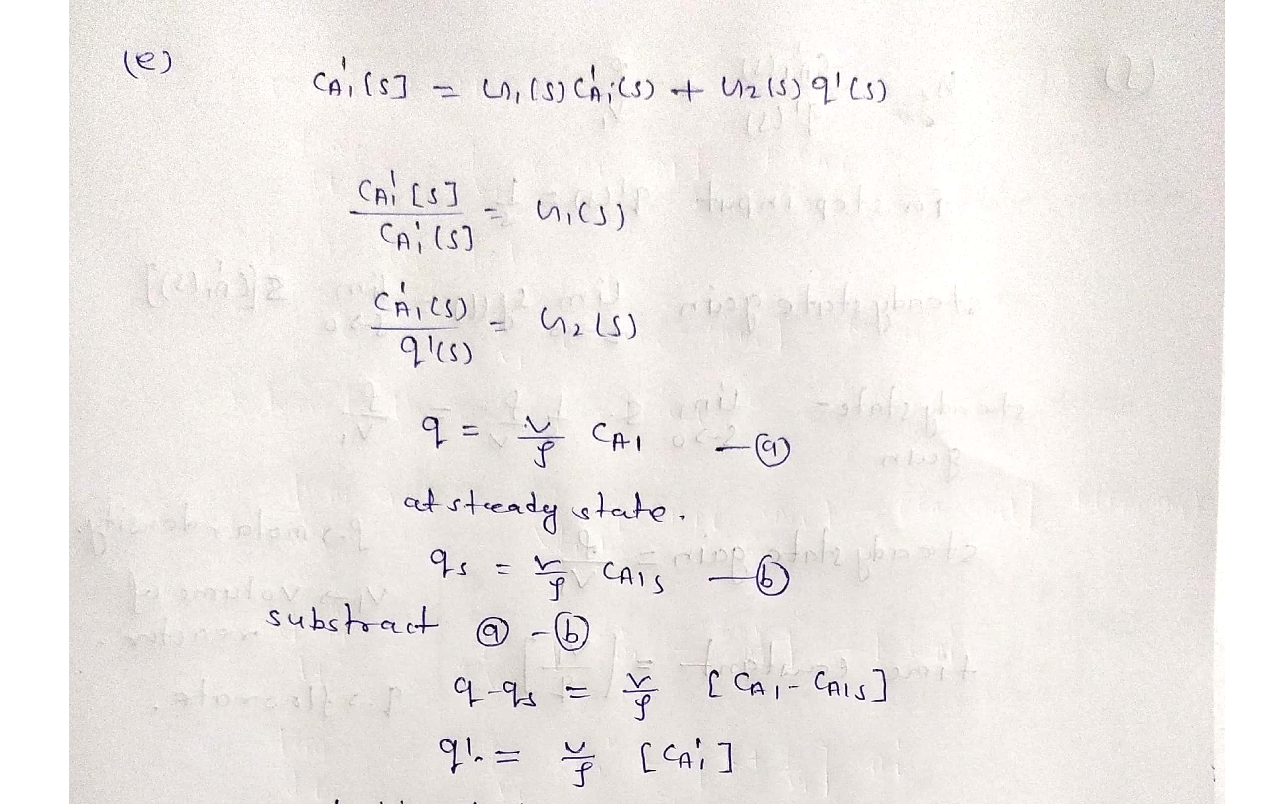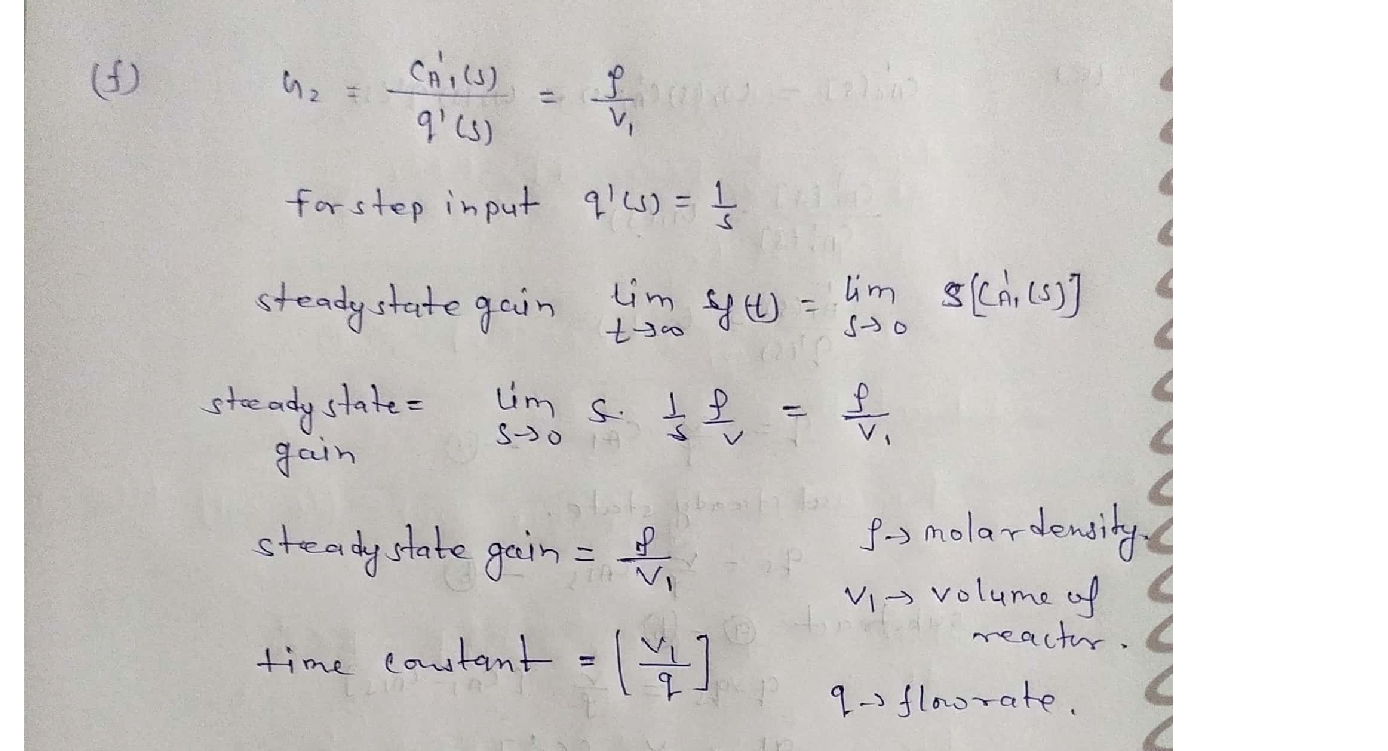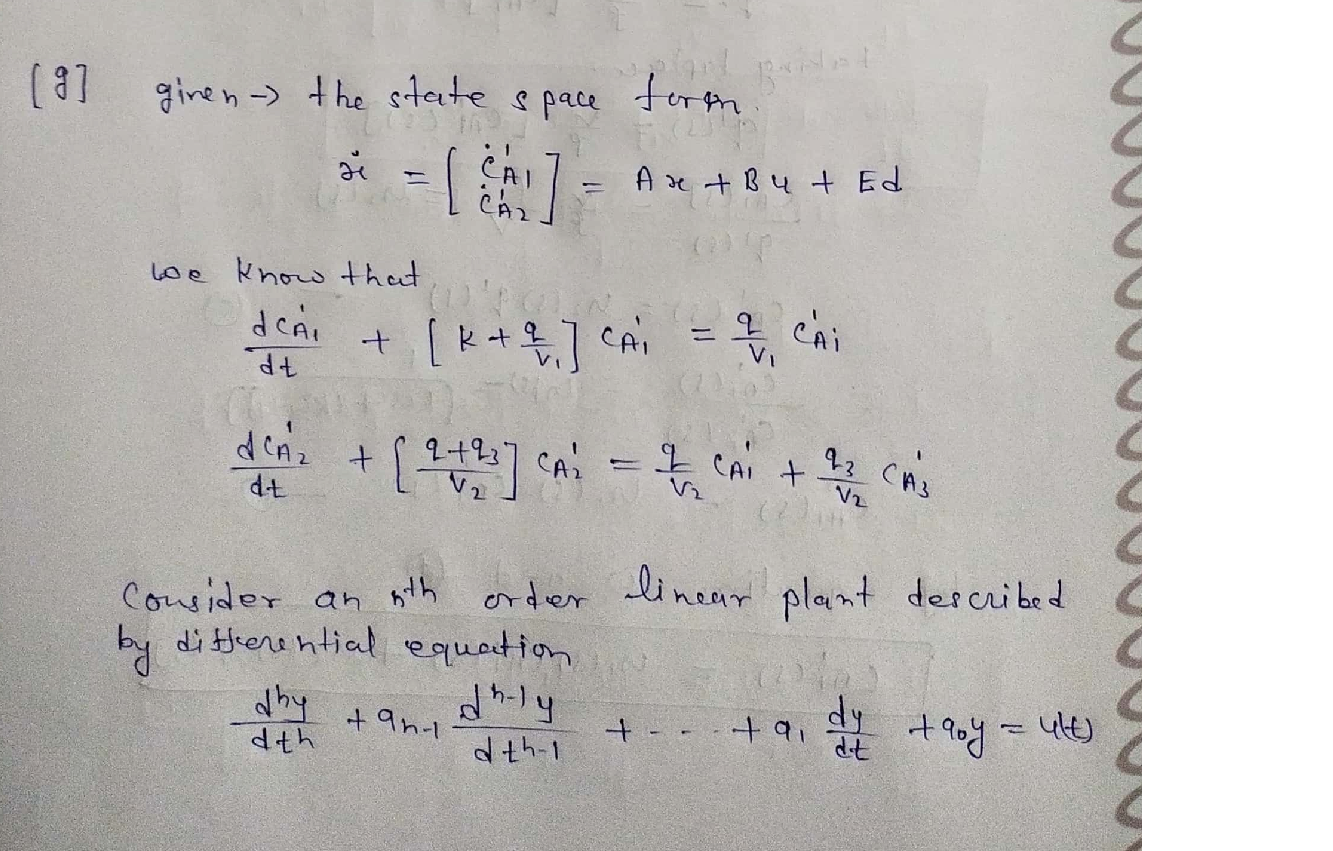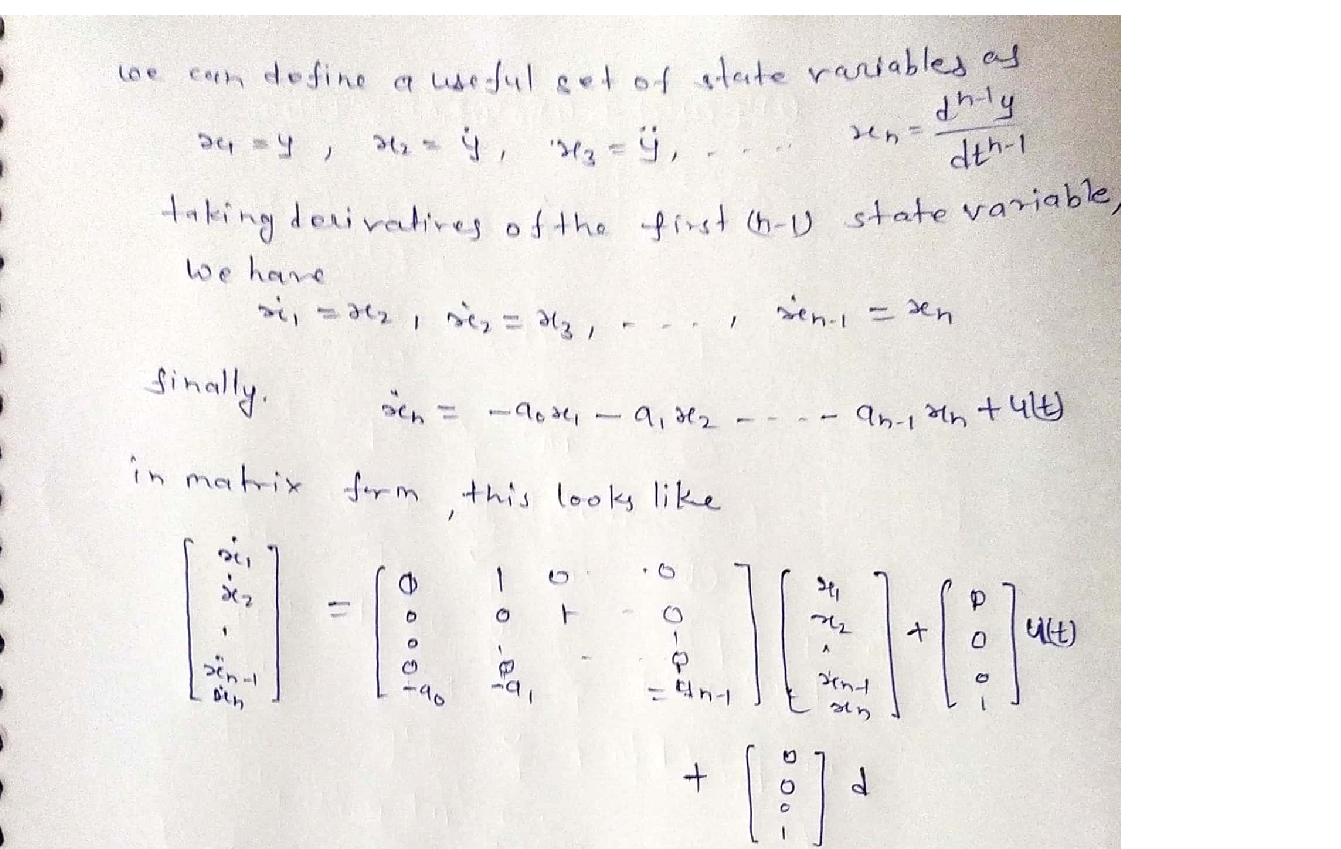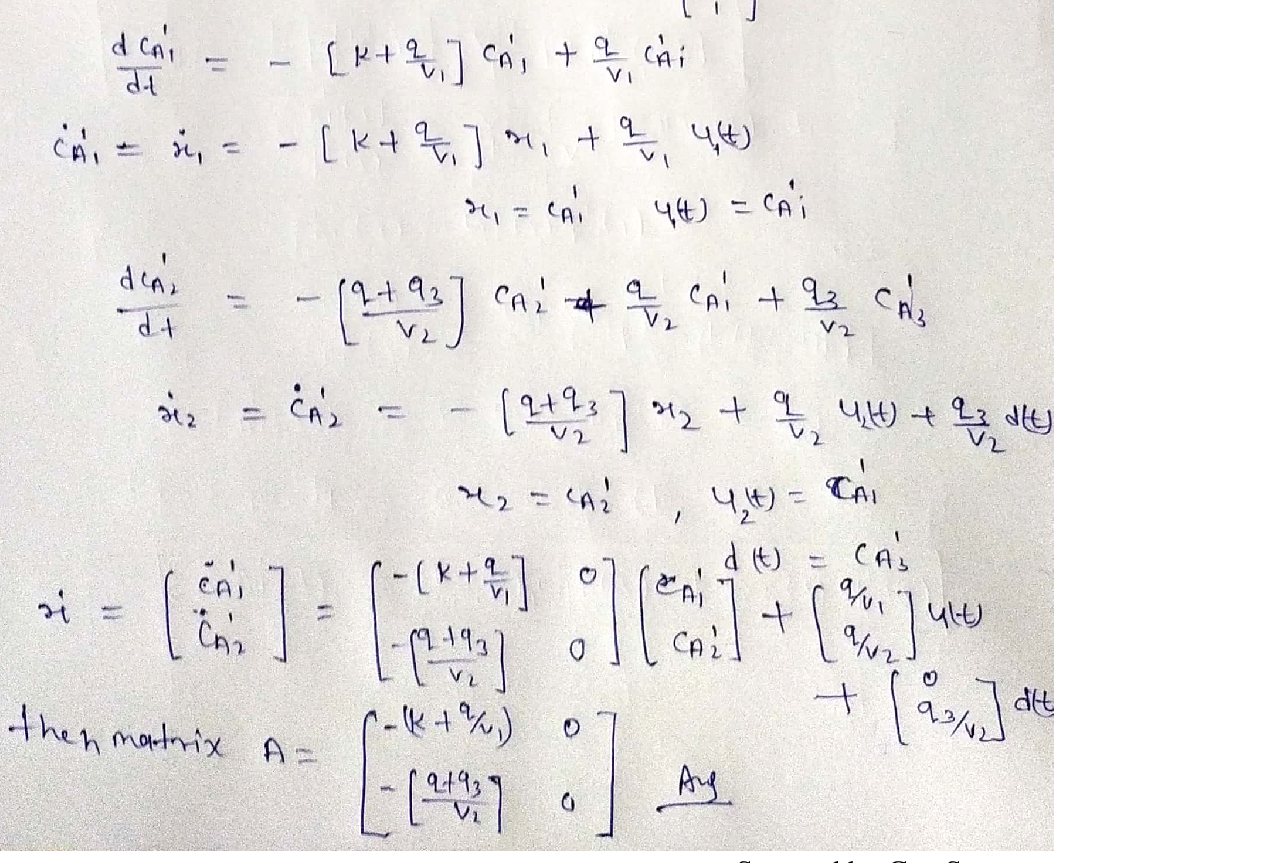### Question 28676Process Control

1. Both tanks are perfectly mixed.
2. Both tanks operate at constant volume, V and V.
3. The fluid density P is constant.
4. In the first tank, component A is consumed according to:
RA = -kCA
where k is a known constant. There is no chemical reaction in the second tank.Write
a. Write dynamic component A balances for both tanks. (Do not write any energy balances.)Eliminate total mass balances (if you wrote them), q2, and the rate equation, so that you onlyhave two equations for:
\frac{d c_{A 2}}{d t}=f_{2}\left(q, q_{3}, c_{A 1}, c_{A 2}, c_{A 3}\right)
b. Perform a degree of freedom analysis on your model. The variables should include:
\frac{d c_{A 1}}{d t}=f_{1}\left(q, c_{A i}, c_{A 1}\right)
q, q_{3}, c_{A i}, c_{A 1}, c_{A 2}, c_{A 3} \text {. Determine the model inputs and model outputs. Also assign DVs }
and MVs.
c. Linearize your model equations, and convert them to perturbation variables.
d. Take the Laplace transform of the linearized equation for dc,, (t)/dt from part c. You do not need to take the Laplace transform of the equation for dc', (t)/dtAlA2
e. Show that:
c_{A 1}^{\prime}(s)=G_{1}(s) c_{A i}^{\prime}(s)+G_{2}(s) q^{\prime}(s)
f. For G2 (s), find the steady-state gain and all time constants.
g. In part c, you linearized the model equations. These can be written in the state-space form:
\dot{x}=\left[\begin{array}{l} \dot{c}_{A 1}^{\prime} \\ \dot{c}_{A 2}^{\prime} \end{array}\right]=A x+B u+E d
Find the matrix A.

### Submit query

Getting answers to your urgent problems is simple. Submit your query in the given box and get answers Instantly.

### Submit a new QuerySuccess

Assignment is successfully created